Question
Find the actual interest rate paid, to the nearest tenth, on the simple discount note.
\$1000; discount rate 7.8%; length of loan 6 mo
A) 10.1%
B) 9.1%
C) 7.1%
D) 8.1%

We need at least 10 more requests to produce the answer.

0 / 10 have requested this problem solution

The more requests, the faster the answer.

All students who have requested the answer will be notified once they are available.

#### Earn Coins

Coins can be redeemed for fabulous gifts.

Similar Homework Help Questions
• ### Please help me with these two problems: Find the actual interest paid, to the nearest tenth, on the simple discount note

Please help me with these two problems: Find the actual interest paid, to the nearest tenth, on the simple discount note. \$27,000; discount rate 7%; length of loan 3 mo. \$50,000; discount rate 8%; length of loan 6 mo.

• ### Sam Peters needs to calculate the effective interest rate of a simple discount note for \$3,600,...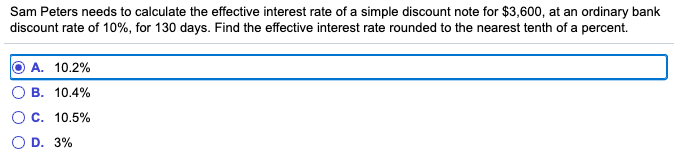Sam Peters needs to calculate the effective interest rate of a simple discount note for \$3,600, at an ordinary bank discount rate of 10%, for 130 days. Find the effective interest rate rounded to the nearest tenth of a percent. O A. 10.2% OB. 10.4% O C. 10.5% OD. 3%

• ### A 3-month \$25,000 treasury bill with a simple annual discount rate of 0.25% was sold in...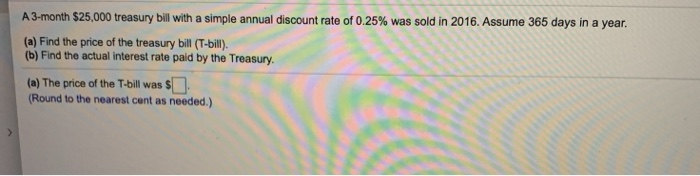A 3-month \$25,000 treasury bill with a simple annual discount rate of 0.25% was sold in 2016. Assume 365 days in a year. (a) Find the price of the treasury bill (T-bill). (b) Find the actual interest rate paid by the Treasury. (a) The price of the T-bill was \$] (Round to the nearest cent as needed.) A 6-month \$20,500 treasury bill with a simple annual discount rate of 0.44% was sold in 2016. Assume 365 days in a year....

• ### Business math: simple interest question

You were offered either a simple interest note or a simple discount note with the following terms: \$33,264 at 8% for 18 months. Calculate the effective interest rate. (Do not round intermediate calculations. Round your final answer to the nearest tenth percent.) Please help I have been trying to do this problem for an hour and I can't find the correct answer

• ### Bill Blank signed an \$7,540 note at Citizen's Bank. Citizen's charges a 8.2% discount rate. Assume...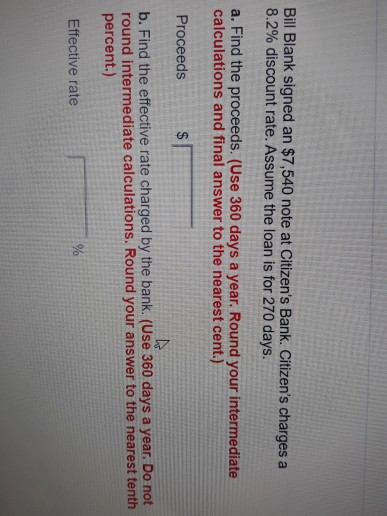Bill Blank signed an \$7,540 note at Citizen's Bank. Citizen's charges a 8.2% discount rate. Assume the loan is for 270 days. a. Find the proceeds. (Use 360 days a year. Round your intermediate calculations and final answer to the nearest cent.) Proceeds b. Find the effective rate charged by the bank. (Use 360 days a year. Do not round intermediate calculations. Round your answer to the nearest tenth percent.) Effective rate You were offered the opportunity to purchase either...

• ### The face value of a simple discount note is \$4,000. The bank discount is calculated at...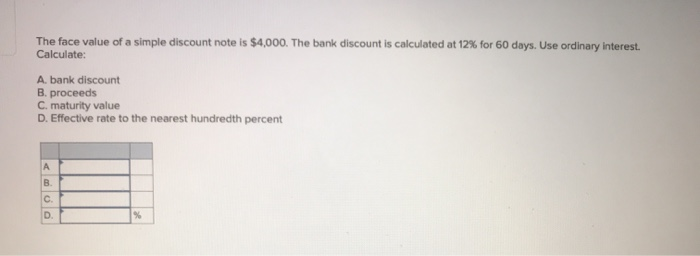The face value of a simple discount note is \$4,000. The bank discount is calculated at 12% for 60 days. Use ordinary interest. Calculate: A bank discount B. proceeds C. maturity value D. Effective rate to the nearest hundredth percent

• ### find the interest paid on loan for 2 years simple interest rate of 9% per year

find the interest paid on loan for 2 years simple interest rate of 9% per year

• ### Find the interest rate on a loan charging \$765 simple interest on a principal of \$3750...

Find the interest rate on a loan charging \$765 simple interest on a principal of \$3750 after 6 years. __________ Find the simple interest on the loan. \$1900 at 8% for 10 years. _________ Find the term of a loan of \$225 at 3.5% if the simple interest is \$63. __________ Determine the amount due on the compound interest loan. (Round your answers to the nearest cent.) \$16,000 at 4% for 15 years if the interest is compounded in the...

• ### none of my answes match the answers given for these questions. Find the exact interest. Use...none of my answes match the answers given for these questions. Find the exact interest. Use 365 days in a year, and use the exact number of days in a month. Assume simple interest and discount. Round to the nearest cent, if necessary. 2) A loan of \$180,000.00 at 7.5% made on June 1 and due on August 21. A) \$2,995.89 B) \$3,037.50 C) \$3,136.13 D) \$3,375.00 The given Treasury bill was sold in August 2008. Find (1) the price...

• ### question 6,7 6. (4 marks) A 182-day promissory note for \$1000 with simple interest at 9%...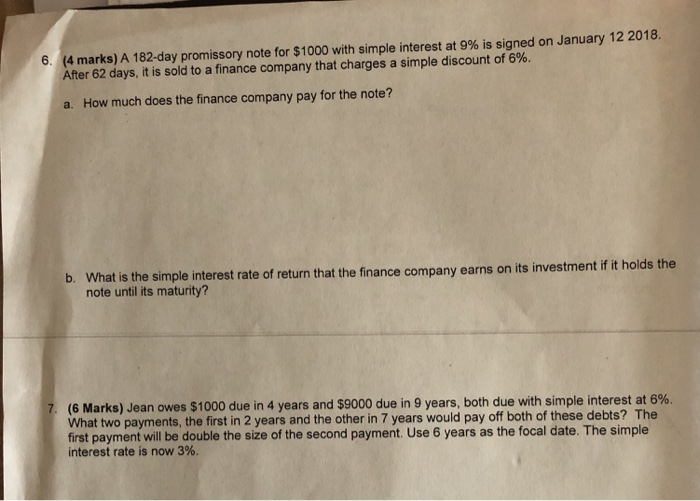question 6,7 6. (4 marks) A 182-day promissory note for \$1000 with simple interest at 9% is signed on January 12 2018. After 62 days, it is sold to a finance company that charges a simple discount of 6%. a. How much does the finance company pay for the note? b. What is the simple interest rate of return that the finance company earns on its investment if it holds the note until its maturity? 7. (6 Marks) Jean owes...